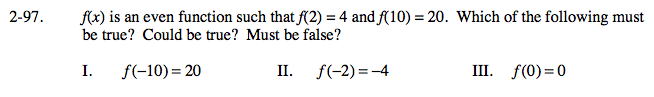### Home > CALC > Chapter Ch2 > Lesson 2.2.4 > Problem2-97

2-97.Even functions have reflective symmetry across the y-axis.

Definition of an even function: f(a) = f(−a)

Must all even functions go through the origin?
Consider the even function: f(x) = x2 + 1.

$\text{Also consider the even function: }f(x)=\frac{1}{x^{2}}$